# What is a Semiconductor? Discuss the effect of temperature on semiconductors. Describe the mechanism of hole current flow in a semiconductor.

## What Is A Semiconductor

A semiconductor is a substance which has resistivity in between conductors and insulators, e.g. germanium,silicon,selenium,carbon etc.

### Properties of Semiconductor

1. The resistivity of a semiconductor is less than an insulator but more than a conductor.
2. It has negative temperature co-efficient of resistance. That means the resistance of a semiconductor decreases with increase in temperature and vice-versa.
3. When a suitable metallic impurity is added to a semiconductor, its current conducting properties change appreciably.

### Commonly Used Semiconductors

The two most frequently used semiconductors are (i) germanium (Ge) and (ii) silicon (Si).

It is because the energy required to break their co-valent bonds is very small; being 0.7 eV for Ge and 1.1 eV for Si.

#### (i) Germanium(Ge)

Atomic number of germanium is 32. So it has 32 protons and 32 electrons.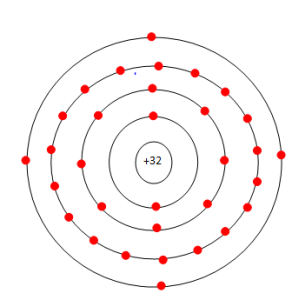Fig.1 (i)Fig.1 (ii)

Two electrons are in the first orbit, eight electrons are in the second, eighteen electrons in the third and four electrons in the outer or valence orbit. This is shown in fig.1(i).

It is clear that germanium atom has four valence electrons i.e. it is a tetravalent element.

Fig.1 (ii) shows how the various germanium atoms are held through co-valent bonds.

#### (ii) Silicon(Si)

Atomic number of silicon is 14. So it has 14 protons and 14 electrons.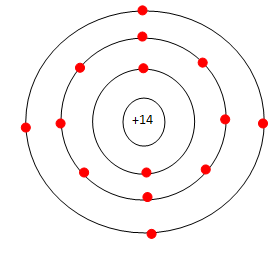Fig.2 (i)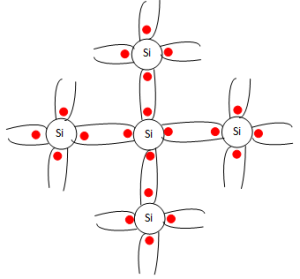Fig.2 (ii)

Two electrons are in the first orbit,eight electrons are in the second orbit and four electrons in the third orbit. This is shown in fig.2(i).

It is clear that silicon atom has four valence electrons i.e. it is a tetravalent element.

Fig.2(ii) shows how various silicon atoms are held through co-valent bonds.

### Energy Band Description of Semiconductor

A semiconductor can be defined much more comprehensively on the basis of energy bands as under: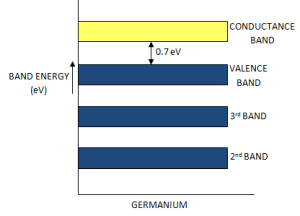Fig.3(i)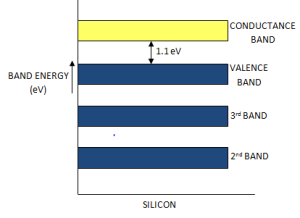Fig.3(ii)

A semiconductor is a substance which has almost filled valence band and nearly empty conduction band with a very small energy gap (nearly equal to 1 eV ) separating the two.

Fig.3 (i) and 3 (ii) shows the energy band diagrams of germanium and silicon respectively.

As the forbidden gap is very small; being 0.7 eV for Ge and 1.1 eV for Si, therefore, relatively small energy is needed by their valence electrons to cross over to the conduction band.

Even at room temperature, some of the valence electrons may acquire sufficient energy to enter into the conduction band and thus become free electrons.

However, at this temperature, the number of free electrons available is very small. Hence, at room temperature, a piece of Ge or Si is neither a good conductor nor an insulator. For this reason, such substances are called semiconductors.

### Effect of Temperature on Semiconductors

The electrical conductivity of a semiconductor changes appreciably with temperature variations.

#### (i) At absolute zero:

At absolute zero temperature, all the electrons are tightly held by the semiconductor atoms. The inner orbit electrons are bound whereas the valence electrons are engaged in co-valent bonding.

At this temperature, the co-valent bonds are very strong and there are no free electrons. Therefore, the semiconductor behaves as a perfect insulator. This is shown in fig.4(i).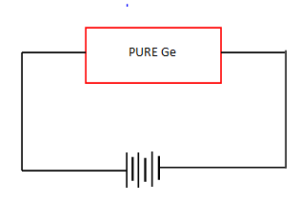Fig.4(i)Fig.4(ii)

In terms of energy band description, the valence band is filled and there is a large energy gap between valence band and conduction band.

Therefore, no valence electrons can reach the conduction band to become free electron.

Hence, the semiconductor behaves as an insulator due to the non-availability of free electrons.

#### (ii) Above absolute zero:

When the temperature is raised, some of the co-valent bonds in the semiconductor break due to the thermal energy supplied.

The breaking of bonds set those electrons free which are engaged in the formation of these bonds.

These free electrons constitute a tiny electric current if potential difference is applied across the semiconductor. This is shown in fig.5(i).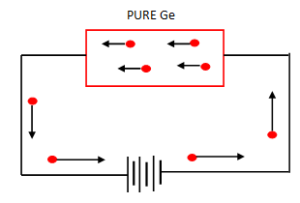Fig.5 (i)

This shows that the resistance of a semiconductor decreases with the increase in temperature i.e. it has negative temperature co-efficient of resistance

Fig.5(ii) shows the energy band diagram.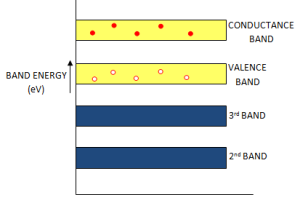Fig.5 (ii)

As the temperature is raised, some of the valence electrons acquire sufficient energy to enter into the conduction band and thus become free electrons.

Under the influence of electric field, these free electrons will constitute electric current.

It may be noted that each time a valence electron enters into the conduction band, a hole is created in the valence band.

### Hole Current

At room temperature, some of the co-valent bonds in pure semiconductor break, setting up free electrons.Under the influence of  electric field, these free electrons constitute electric current.

At the same time, another current;hole current, also flows in the semiconductor.

When a covalent bond is broken due to thermal energy, the removal of one electron leaves a vacancy i.e. a missing electron in the covalent bond. This missing electron is called a hole which acts as a positive charge.

For one electron set free, one hole is created. Therefore, thermal energy creates hole-electron pairs. That means number of  free electrons is equal to number of holes.

The current conduction by holes can be explained as follows: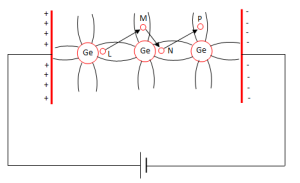Fig.6

Suppose the valence electron at L ,in fig.6 has become free electron due to thermal energy.

This creates a hole in the co-valent bond a L.

The hole is a strong centre of attraction for the electron.

A valence electron at M from nearby co-valent bond comes to fill in the hole at L.

This results in the creation of hole at M.

Another valence electron at N in turn may leaves its bond to fill the hole at M, thus creating a hole at N.

Thus the hole having a positive charge has moved from L to N i.e. towards the negative terminal of supply.

This constitutes hole current.

#### Energy Band Description:

The hole current can be explained in terms of energy bands.

Suppose due to thermal energy, an electron leaves the valence band to enter into the conduction band as shown in fig.7.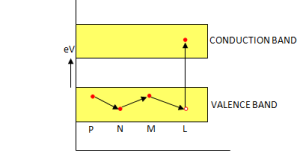Fig.7

This leaves a vacancy at L. Now the valence electron at M comes to fill the hole at L. The result is that hole disappears at L and appears at M.

Next, the valence electron at N moves into the hole at M. Consequently, hole is created at N.

It is now clear  that valence electrons move along the path PNML whereas holes move in the opposite direction i.e. along the path LMNP.